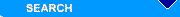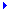Steve's DIY Dyno Welcome to my bit of the webWednesday, 01 April 2020
Miscellaneous
Most hit pages

 HomeDyno Math

 Dyno MathSome of the math used to obatain a power number from an accelerating drum.

# Dynamometer Math

At this stage I wont include the analogies between linear and rotational physics. I may some day include them, but to get this page up and running I'll leave them out for now. You'll just have to take for granted that what I include is correct. (Doesn't necessarily mean that it is though)

#### Symbols:

These symbols aren't entirely correct either, until I find the correct character set they'll have to do for now.

``````
I = rotational inertia

F = force

L = length (i.e. thickness) of inertia wheel

m = mass

P = power

RPM = revolutions per minute

t = time

t = torque

a = angular acceleration

P = ratio between the circumference of a circle and its diameter, roughly 3.14159

w = angular velocity
```
```

#### Re-cap of the physics:

An Inertial Dynamometer, measures how quickly a given engine can accelerate a known rotational inertia from one rpm to another. This is enough information to know the Torque the engine produced during that time, and, since we already know the rpm, the Power the engine produced during that time. With enough steps in rpm, this produces a smooth curve of Torque versus RPM and Power versus RPM.

Newton's Second Law says that Force = mass times acceleration,
F = m * a (A linear anology that I couldn't leave out.)

The relationship for rotational motion is; Torque (t) = Rotational Inertia (I) times Angular acceleration (a)
t = I * a

#### How do you measure Rotational Inertia (I)?

There are a few formulae for working out the moment of inertia of differently constructed drums / rollers. I'll only deal with the one here. The formula for a solid cylindrical drum / roller made of uniform material is;
I = m/2 * r2

You can calculate the mass of the drum or other components by calculating the volume of the piece and using the density figure i.e. 7900kg / m3 for steel.

Using the dimensions of my drum, I'll calculate it's MOI here.

The drum is 360mm wide, has a diameter of 460mm and has 2 stub axles 120mm X 80mm.

Volume for a cylinder = Pr2* h

V = P * .230m2 * .360m
V = 0.0598284 m3 * 7900 (density kg/m3) = 472.6450kg

Now for the Stub axles;
V = P * .040m2 * .120m
V = 0.0598284 m3 * 7900 (density kg/m3) = 1.5168kg

Now for the MOI
I = m/2 * r2

The drum;
I = 472.6450/2 * .2302
I = 12.5014kg×m2

The stub axles;
I = 1.5168/2 * .1202
I = 0.02184kg×m2 now times 2 there are two of them!

Grand Total of;
MOI = 12.5451kg×m2

(I had my drum weighed and it came up at 476kg, the calculated weight is 475.6786kg. Good enough for me.)

(By the way, notice how much the radius of the cylinder affects its rotational inertia. First of all, the mass depends on the radius squared. A cylinder twice the diameter of another has four times the mass. Then, the rotational inertia is that times radius squared AGAIN. This comes from the particle distribution. Two cylinders with the same mass, but one with twice the radius of the other (i.e. not so wide, but with a bigger diameter), has four times the rotational inertia. This is why I settled on the largest diameter I could get hold of.)

#### Acceleration

a = Dw / Dt     Angular acceleration (a) equals change in angular velocity (w) per change in time.

For the formula to work, the acceleration has to be in radians/second/second. (rad/sec2). There are 2Prads in one revolution.

Angular velocity (w) is simply how quickly something turns, like RPM, or Revolutions per Minute. To keep the units consistent, you want to use radians per second (1 revolution = 6.28 radians (2P) before you plug it into any calculation, but it's easier to think of RPM. If you go from say, 5,000 rpm to 10,000 rpm in five seconds, the Angular Acceleration is 1,000 rpm per second. My front-end program only works in rads/sec, then I convert these to rpm's where needed.

#### Putting it all together

Here I'll plug in some figures that come from my data acquisition module and step through the manipulation of these figures to get a torque and power value for this particular sample. These two values actually came from a run I did with my TL1000S. When you have a couple of hundred of these samples and plot then, this results as a pretty reasonable graph.

The module transmits hexadecimal values (hex speeds up the module processing time) so the first step is to change these to decimal.
Sample 1, H173D = 5949
Sample 2, H1731 = 5937

What are these numbers? Each one is how long the drum took to do half a revolution in 2 microsecond (ms) increments. So now we have,
Sample 1, 11898ms
Sample 2, 11874ms

I'll move straight onto power now, I'll finish the acceleration calculations off one day.

#### Power

When we know how much torque is being applied to the drum, and we know the drum speed in revolutions per minute (rpm) or Rads per second (Rad/s) we can calulate how much work is being done or how much power is being produced.

P = t * w (Power = torque by angular velocity).

I'll make up some values and plug them into the formula.
t = 358

P = 358 * 209 = 74,822 watts
74,822 / 1000 = 74.822 Kilowatts (Kw).

(1 watt = 1 Joule / sec, like I said, some of the information supplied will have to be taken for granted as being correct so I don't have to break every bit down into how it is obtained or worked out.)

Converting kilowatts to horsepower or metric horsepower (pferdestarke = ps) is just a simple matter of multiplying the answer by a constant.

Kw to Hp multiply by 1.34
Kw to Metric HP (ps) multiply by 1.35962

So 74.822Kw * 1.34 = 100.3HP

Note, these figures are at the drum, so the bike made 100hp, but the 2000rpm was the drum RPM, the torque was the value seen by the drum. The bike RPM may have been 8000rpm which would make the bike engine torque 89.5

#### Simplified formula

Have you seen the simplified formulae for Power and Torque figures? For you imperial guys the torque and power curves will always cross at the 5252rpm mark. That is if your engine is making 60hp @ 5252rpm then it is making 60ft/lbs of torque at that point too.

It's a bit harder for the metric guys, the power and torque curves cross at the 9549rpm mark. That is if you are making 80KW @ 9549rpm then the engine is making 80Nm of torque at that point too.

Horsepower = (Torque * RPM)/5252

Why?
It's important to understand that nobody on the planet ever actually measures horsepower from a running engine. What we actually measure (on a dynomometer) is torque, expressed in foot pounds (in the U.S.), and then we *calculate* actual horsepower by converting the twisting force of torque into the work units of horsepower.

Visualize a one pound weight, one foot from the fulcrum on a weightless bar. If we rotate that weight for one full revolution against a one pound resistance, we have moved it a total of 6.2832 feet (Pi * a two foot circle), and, incidently, we have done 6.2832 foot pounds of work.

Remember Watt? He said that 33,000 foot pounds of work per minute was equivalent to one horsepower. If we divide the 6.2832 foot pounds of work we've done per revolution of that weight into 33,000 foot pounds, we come up with the fact that one foot pound of torque at 5252 rpm is equal to 33,000 foot pounds per minute of work, and is the equivalent of one horsepower. If we only move that weight at the rate of 2626 rpm, it's the equivalent of 1/2 horsepower (16,500 foot pounds per minute), and so on. Therefore, the formula applies for calculating horsepower from a torque measurement.

Kilowatts = (Torque * RPM) / 9549

It's much simpler to work out how 9549 constant came to being for the metric one. We need to convert RPM to Rad/s, and the Power is Kilowatts not just Watts, so there is the difference of 1000 times as well. So 1 rpm / 60 = rps = 0.01666 * 2PRad = 0.1047 Now 1000/0.1047 = 9549.29

One day I may finish this off!